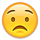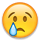# Server-side checkbox filter

edited November 2018

Hello, this is my table with 2 checkboxes: live.datatables.net/xasihece/1/edit

I'm trying to filter data in my table with these two checkboxes (will be more checkboxes and date-picker in the future). I'm using the php server_processing php and ssp.class.php. I found some comments about custom filtering in the ssp.class.php file, but there is no example how to do it and there is no example also in the DataTables forum or internet.

I know that I need to modify the WHERE conditoin in the ssp.class.php when checkbox is selected, but I'm completely lost how to do it. Thank you in advance.

## Answers

• Posts: 5,619Questions: 0Answers: 981

Hi @culter ,

This thread here should sort you out, it's asking the same thing,

Cheers,

Colin

• Thank you, Colin, the thread is very helpful. But still if I may ask you some questions..

I'm sending the filter state from index.php as this:

``````    <input type="checkbox" id="filter1" name="open" value="1">Open<br>
<script>
\$(document).ready(function() {
var table = \$('#example').DataTable( {
"ajax": {
"url": "scripts/server_processing.php",
"data": function ( d ) {
d.mojaF = \$('#filter1').val();
console.log(d.mojaF);
}
});
});
</script>
``````

I have read in the ssp.class.php comments, that I need to use the complex function to add additional 'where' conditions to the SQL queries, so I changed the

``````echo json_encode(
SSP::simple( \$_GET, \$sql_details, \$table, \$primaryKey, \$columns )
);
``````

to

``````echo json_encode(
SSP::complex( \$_GET, \$sql_details, \$table, \$primaryKey, \$columns )
);
``````

Do I need to apply more changes to server_processing.php or just this one?

Next, as it is written in the ssp.class.php before the complex function I think I need to modify 'All condition' form, but I have no idea how to do it. Can you provide some example, please? Thank you.

• Yes, you need to actually pass a `where` condition as well. It isn't currently doing anything with your `mojaF` variable.

If you look at the code for the SSP class you will see that there are two additional optional arguments for the `complex` method, which can be used to set the condition.

Allan

• Thank you, Allan. I'm sorry, but I have another two questions:

Is it possible to check if the mojaF variable is sent to server_processing script?

Could you please specify how to set the condition in one of the optional arguments? Or is there some example? I prefer to use the \$whereAll, because I will need to restrict records by login id in the future.

Thank you!

• You can use PHP's `isset` method for that - e.g.:

``````\$whereAll = [];
if ( isset( \$_GET['majoF'] ) ) {
\$whereAll = [
"fieldName" => \$_GET['majoF']
];
}
``````

Allan

• Thanks Allan, I have added the isset method to the 'complex' function in ssp.class.php, but I'm not sure if it's the right place. Could you please confirm? And the fieldName is the column name?

I have set the mojaF right in the "data": function (d) { d.mojaF = 1; } for testing purposes, but all I got is "No data available in table" with no error``````static function complex ( \$request, \$conn, \$table, \$primaryKey, \$columns, \$whereResult=null, \$whereAll=null )
{
\$bindings = array();
\$db = self::db( \$conn );
\$localWhereResult = array();
\$localWhereAll = array();
\$whereAllSql = '';
// Build the SQL query string from the request
\$limit = self::limit( \$request, \$columns );
\$order = self::order( \$request, \$columns );
\$where = self::filter( \$request, \$columns, \$bindings );
\$whereResult = self::_flatten( \$whereResult );
\$whereAll = self::_flatten( \$whereAll );

// MY FILTER to filter status column (possible values 0,1)
\$whereAll = [];
if ( isset( \$_GET['mojaF'] ) ) {
\$whereAll = [
"STATUS" => \$_GET['mojaF']
];
}

if ( \$whereResult ) {
\$where = \$where ?
\$where .' AND '.\$whereResult :
'WHERE '.\$whereResult;
}
if ( \$whereAll ) {
\$where = \$where ?
\$where .' AND '.\$whereAll :
'WHERE '.\$whereAll;
\$whereAllSql = 'WHERE '.\$whereAll;
}
.
.
.
``````

Thank you, I appreciate your help.

• I have corrected my code and now the error message is:

DataTables warning: table id=example - An SQL error occurred: SQLSTATE[42S22]: Column not found: 1054 Unknown column 'Array' in 'where clause'

Why it is looking for 'Array' column?

• In Apache's error log is this php notice:

[Mon Nov 26 13:59:20.262555 2018] [:error] [pid 5993] [client 10.10.10.1:50541] PHP Notice: Array to string conversion in /var/www/html/scripts/ssp.class.php on line 316, referer: http://10.10.10.12

• Ok, I was able to set the filter in ssp.class.php as follows:

`````` if ( isset( \$_GET['mojaF'] )) {
\$aab = \$_GET['mojaF'];
\$whereAll = "STATUS IN (\$aab)"; // => \$_GET['mojaF'];
}
``````

It is connected with the d.mojaF in the index.php as follows:

``````Checkbox1: <input type="checkbox" id="testCh" onclick="myCheckFunc()">
<
<script>
function myCheckFunc() {
var checkBox = document.getElementById("testCh");
var text = document.getElementById("textTD");
if(checkBox.checked == true){
text.style.display = "block";
console.log("Checked1: " + checkBox.checked);
var check01 = 1;
} else {
text.style.display = "none";
}
}

\$(document).ready(function() {
var table = \$('#example').DataTable( {
"processing": true,
"serverSide": true,
"responsive": true,
"keys": true,
"language": { "processing": "Hang on. Waiting for data.." },
"ajax": {
"url": "scripts/server_processing.php",
"data": function ( d ) {
d.mojaF = 1;
}
.
.
.
</script>
``````

So when I change d.mojaF variable in the function(d), the sql query will change appropriately, but how to get the checkbox state to the function(d)? Thanks

• I'd actually suggest you do the `isset` in your main script - not in the ssp class - e.g.:

``````\$whereAll = [];
if ( isset( \$_GET['majoF'] ) ) {
\$whereAll = [
"fieldName" => \$_GET['majoF']
];
}

echo json_encode(
SSP::complex( \$_GET, \$sql_details, \$table, \$primaryKey, \$columns, null, \$whereAll )
);
``````

Allan

• Thanks, I rewrote it in server_processing.php as follows:

`````` \$whereAll = [];
if (\$_GET['check01'] == 5) {
\$status1 = "0,1,2";
} elseif (\$_GET['check01'] == 0) {
\$status1 = "0";
} elseif (\$_GET['check01'] == 1) {
\$status1 = "1";
} elseif (\$_GET['check01'] == 2) {
\$status1 = "2";
}
\$whereAll = "STATUS IN (\$status1)";

echo json_encode(
SSP::complex( \$_GET, \$sql_details, \$table, \$primaryKey, \$columns, null, \$whereAll )
);
``````

and this is the function for first checkbox and DataTable (index.php):

`````` <script>
var check01 = 5;
function myCheckFunc() {
var checkBox = document.getElementById("testCh");
if(checkBox.checked == true){
check01 = 0;
} else {
check01 = 5;
}
}

\$(document).ready(function() {
var table = \$('#example').DataTable( {
"processing": true,
"serverSide": true,
"responsive": true,
"keys": true,
"language": { "processing": "Hang on. Waiting for data.." },
"ajax": {
"url": "scripts/server_processing.php"
"data": function ( d ) {
d.check01 = check01; }
.
.
.
``````

</script>

When I change the value of check01 (2nd script, line 2) manually, it is working fine and the table displays filtered data, but when I click on the checkbox, the table doesn't refresh with the filtered data and no change is visible.

• In `myCheckFunc()` are you wanting the Datatables to be refreshed? If so you can try either `draw()` or `ajax.reload()` to send a request to the server fro fresh data.

Kevin

• Thanks Kevin, I have posted complete code of myCheckFunc(), so I'm not waiting for the DataTables refresh. Recently I tried to use ajax.reload() as follows

`````` table.ajax.reload( function (json) {
\$('#testCh').val( json.lastInput);
} );
``````

but with no effectTomorrow I will try to use draw(). Hope it will work. Thank you.

• Ok,

`\$('#example').DataTable().ajax.reload();`

is working and the table is redrawn, but nothing changed, because the variable check01 from myCheckFunc() did'nt get to dataTableMy code is as follows:

``````Checkbox1: <input type="checkbox" id="testCh" onclick="myCheckFunc()">

<script>
var check01 = 5;      // when I change this variable to 0, it's working and data are filtered
// CHECKBOX FUNCTION
function myCheckFunc() {
var checkBox = document.getElementById("testCh");
if(checkBox.checked == true){
var check01 = 0;
console.log("check01: " + check01);      // the variable change it's value from 5 to 0 correctly
\$('#example').DataTable().ajax.reload();
} else {
check01 = 5;
console.log("check01: " + check01);
}
}

\$(document).ready(function() {
var table = \$('#example').DataTable( {
"processing": true,
"serverSide": true,
"responsive": true,
"keys": true,
"language": { "processing": "Hang on. Waiting for data.." },
"ajax": {
"url": "scripts/server_processing.php",
"data": function ( d ) {
d.check01 = check01;
// Here is the problem, following returns 5 no matter if the checkbox is checked or not :(
console.log("f_d.check01: " + d.check01);
.
.
.
``````

The myCheckFunc() is out of DataTable(). Maybe it's problem with local/global variables. I tried to add the myCheckFunc() in the \$(document.ready(function() {}, but I've got and error, that myCheckFunction in line 1 is not definedDo you have some ide how to get the value of check01 variable to the function in DataTable?

Thank you

• In line 9 you have `var check01 = 0;`. Remove the `var` part. This is defining a local scope variable for the `myCheckFunc` function.

Kevin

• I owe you 2 beers now. Fantastic! I have my first checkbox filter, many thanks to all of you. Now the other 10 checkboxes and it's done.Have a nice day.

• Hello, Culter This is exactly what I'm looking. I was trying to create checkbox filter using serverside but its not working this one

Can you share me the complete codes of your custom filter from the checkbox to js and serverside.php please• Posts: 5,619Questions: 0Answers: 981

Hi @hashsalacop ,

We're happy to take a look, but as per the forum rules, please link to a test case - a test case that replicates the issue will ensure you'll get a quick and accurate response. Information on how to create a test case (if you aren't able to link to the page you are working on) is available here.

Cheers,

Colin

• Hi colin here is my sample but i dont know how to work the serverside.php on this.this are the just a simple code on my data the javascript and the html. this one

• Posts: 5,619Questions: 0Answers: 981

Hi @hashsalacop ,

The problem is that you're using regular expressions, which the supplied server-side scripts don't support. This thread here has some discussion on it.

Cheers,

Colin

• I dont really get it.. i was trying to solve this for 2 weeks and up until now i can't solve it. can you give me a example there is no response on the ajax.

Sign In or Register to comment.